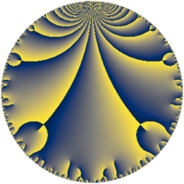# Properties

 Label 70.6.aLevel $70$ Weight $6$ Character orbit 70.a Rep. character $\chi_{70}(1,\cdot)$ Character field $\Q$ Dimension $10$ Newform subspaces $8$ Sturm bound $72$ Trace bound $3$

# Related objects

## Defining parameters

 Level: $$N$$ $$=$$ $$70 = 2 \cdot 5 \cdot 7$$ Weight: $$k$$ $$=$$ $$6$$ Character orbit: $$[\chi]$$ $$=$$ 70.a (trivial) Character field: $$\Q$$ Newform subspaces: $$8$$ Sturm bound: $$72$$ Trace bound: $$3$$ Distinguishing $$T_p$$: $$3$$

## Dimensions

The following table gives the dimensions of various subspaces of $$M_{6}(\Gamma_0(70))$$.

Total New Old
Modular forms 64 10 54
Cusp forms 56 10 46
Eisenstein series 8 0 8

The following table gives the dimensions of the cuspidal new subspaces with specified eigenvalues for the Atkin-Lehner operators and the Fricke involution.

$$2$$$$5$$$$7$$FrickeDim.
$$+$$$$+$$$$+$$$$+$$$$1$$
$$+$$$$+$$$$-$$$$-$$$$1$$
$$+$$$$-$$$$+$$$$-$$$$1$$
$$+$$$$-$$$$-$$$$+$$$$1$$
$$-$$$$+$$$$+$$$$-$$$$2$$
$$-$$$$+$$$$-$$$$+$$$$1$$
$$-$$$$-$$$$+$$$$+$$$$1$$
$$-$$$$-$$$$-$$$$-$$$$2$$
Plus space$$+$$$$4$$
Minus space$$-$$$$6$$

## Trace form

 $$10 q + 8 q^{2} - 44 q^{3} + 160 q^{4} + 16 q^{6} + 128 q^{8} + 986 q^{9} + O(q^{10})$$ $$10 q + 8 q^{2} - 44 q^{3} + 160 q^{4} + 16 q^{6} + 128 q^{8} + 986 q^{9} + 1248 q^{11} - 704 q^{12} - 808 q^{13} - 1100 q^{15} + 2560 q^{16} - 1980 q^{17} + 5800 q^{18} + 1220 q^{19} - 980 q^{21} - 1856 q^{22} - 6024 q^{23} + 256 q^{24} + 6250 q^{25} - 2528 q^{26} + 15400 q^{27} - 3412 q^{29} + 3600 q^{30} + 9928 q^{31} + 2048 q^{32} - 6208 q^{33} - 17072 q^{34} + 2450 q^{35} + 15776 q^{36} - 15508 q^{37} + 17040 q^{38} - 24504 q^{39} + 28412 q^{41} + 7056 q^{42} - 21408 q^{43} + 19968 q^{44} - 11600 q^{45} - 32096 q^{46} - 84296 q^{47} - 11264 q^{48} + 24010 q^{49} + 5000 q^{50} - 4344 q^{51} - 12928 q^{52} + 46228 q^{53} + 61024 q^{54} - 27800 q^{55} - 12000 q^{57} + 19344 q^{58} - 39324 q^{59} - 17600 q^{60} - 62800 q^{61} - 66400 q^{62} - 46256 q^{63} + 40960 q^{64} + 77500 q^{65} + 66240 q^{66} + 151304 q^{67} - 31680 q^{68} - 160624 q^{69} + 9800 q^{70} - 137080 q^{71} + 92800 q^{72} - 52436 q^{73} - 47888 q^{74} - 27500 q^{75} + 19520 q^{76} + 47432 q^{77} - 99296 q^{78} - 17240 q^{79} + 121610 q^{81} + 34096 q^{82} + 302668 q^{83} - 15680 q^{84} + 46600 q^{85} + 2368 q^{86} + 216600 q^{87} - 29696 q^{88} + 216996 q^{89} - 142400 q^{90} - 86828 q^{91} - 96384 q^{92} - 336208 q^{93} - 29280 q^{94} - 109500 q^{95} + 4096 q^{96} + 108340 q^{97} + 19208 q^{98} + 627680 q^{99} + O(q^{100})$$

## Decomposition of $$S_{6}^{\mathrm{new}}(\Gamma_0(70))$$ into newform subspaces

Label Dim. $$A$$ Field CM Traces A-L signs $q$-expansion
$$a_2$$ $$a_3$$ $$a_5$$ $$a_7$$ 2 5 7
70.6.a.a $$1$$ $$11.227$$ $$\Q$$ None $$-4$$ $$-23$$ $$25$$ $$49$$ $$+$$ $$-$$ $$-$$ $$q-4q^{2}-23q^{3}+2^{4}q^{4}+5^{2}q^{5}+92q^{6}+\cdots$$
70.6.a.b $$1$$ $$11.227$$ $$\Q$$ None $$-4$$ $$-9$$ $$25$$ $$-49$$ $$+$$ $$-$$ $$+$$ $$q-4q^{2}-9q^{3}+2^{4}q^{4}+5^{2}q^{5}+6^{2}q^{6}+\cdots$$
70.6.a.c $$1$$ $$11.227$$ $$\Q$$ None $$-4$$ $$-3$$ $$-25$$ $$49$$ $$+$$ $$+$$ $$-$$ $$q-4q^{2}-3q^{3}+2^{4}q^{4}-5^{2}q^{5}+12q^{6}+\cdots$$
70.6.a.d $$1$$ $$11.227$$ $$\Q$$ None $$-4$$ $$11$$ $$-25$$ $$-49$$ $$+$$ $$+$$ $$+$$ $$q-4q^{2}+11q^{3}+2^{4}q^{4}-5^{2}q^{5}-44q^{6}+\cdots$$
70.6.a.e $$1$$ $$11.227$$ $$\Q$$ None $$4$$ $$-17$$ $$25$$ $$-49$$ $$-$$ $$-$$ $$+$$ $$q+4q^{2}-17q^{3}+2^{4}q^{4}+5^{2}q^{5}-68q^{6}+\cdots$$
70.6.a.f $$1$$ $$11.227$$ $$\Q$$ None $$4$$ $$-11$$ $$-25$$ $$49$$ $$-$$ $$+$$ $$-$$ $$q+4q^{2}-11q^{3}+2^{4}q^{4}-5^{2}q^{5}-44q^{6}+\cdots$$
70.6.a.g $$2$$ $$11.227$$ $$\Q(\sqrt{3369})$$ None $$8$$ $$3$$ $$-50$$ $$-98$$ $$-$$ $$+$$ $$+$$ $$q+4q^{2}+(2-\beta )q^{3}+2^{4}q^{4}-5^{2}q^{5}+\cdots$$
70.6.a.h $$2$$ $$11.227$$ $$\Q(\sqrt{1129})$$ None $$8$$ $$5$$ $$50$$ $$98$$ $$-$$ $$-$$ $$-$$ $$q+4q^{2}+(3-\beta )q^{3}+2^{4}q^{4}+5^{2}q^{5}+\cdots$$

## Decomposition of $$S_{6}^{\mathrm{old}}(\Gamma_0(70))$$ into lower level spaces

$$S_{6}^{\mathrm{old}}(\Gamma_0(70)) \cong$$ $$S_{6}^{\mathrm{new}}(\Gamma_0(5))$$$$^{\oplus 4}$$$$\oplus$$$$S_{6}^{\mathrm{new}}(\Gamma_0(7))$$$$^{\oplus 4}$$$$\oplus$$$$S_{6}^{\mathrm{new}}(\Gamma_0(10))$$$$^{\oplus 2}$$$$\oplus$$$$S_{6}^{\mathrm{new}}(\Gamma_0(14))$$$$^{\oplus 2}$$$$\oplus$$$$S_{6}^{\mathrm{new}}(\Gamma_0(35))$$$$^{\oplus 2}$$